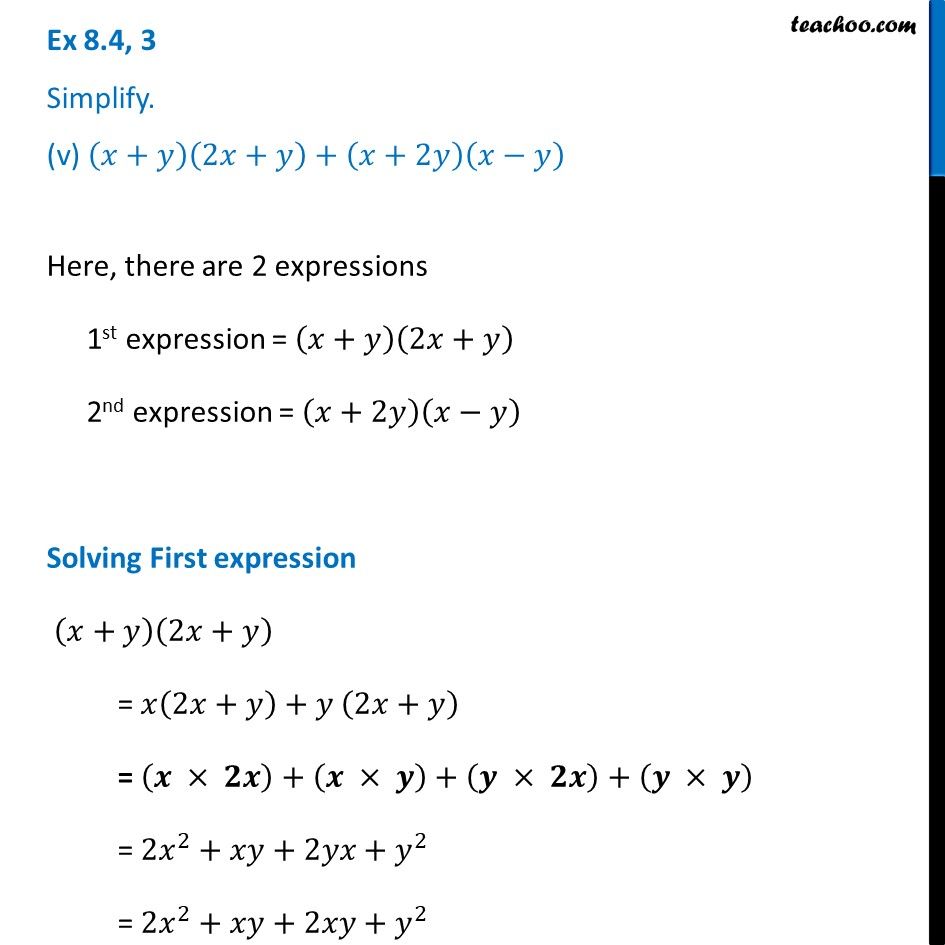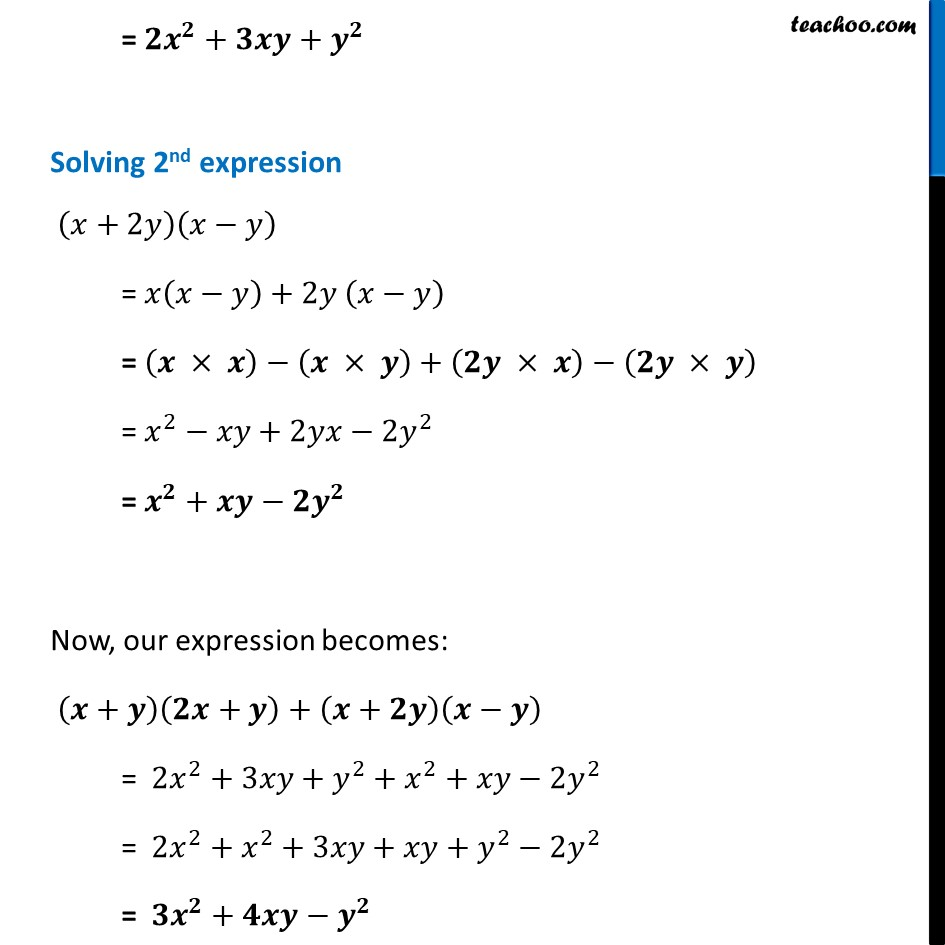Ex 8.4

Chapter 8 Class 8 Algebraic Expressions and Identities
Serial order wiseLearn in your speed, with individual attention - Teachoo Maths 1-on-1 Class

### Transcript

Ex 8.4, 3 Simplify. (v) (𝑥+𝑦)(2𝑥+𝑦)+(𝑥+2𝑦)(𝑥−𝑦)Here, there are 2 expressions 1st expression = (𝑥+𝑦)(2𝑥+𝑦) 2nd expression = (𝑥+2𝑦)(𝑥−𝑦) Solving First expression (𝑥+𝑦)(2𝑥+𝑦) = 𝑥(2𝑥+𝑦)+𝑦 (2𝑥+𝑦) = (𝒙 × 𝟐𝒙)+(𝒙 × 𝒚)+(𝒚 × 𝟐𝒙)+(𝒚 × 𝒚) = 2𝑥^2+𝑥𝑦+2𝑦𝑥+𝑦^2 = 2𝑥^2+𝑥𝑦+2𝑥𝑦+𝑦^2 = 𝟐𝒙^𝟐+𝟑𝒙𝒚+𝒚^𝟐 Solving 2nd expression (𝑥+2𝑦)(𝑥−𝑦) = 𝑥(𝑥−𝑦)+2𝑦 (𝑥−𝑦) = (𝒙 × 𝒙)−(𝒙 × 𝒚)+(𝟐𝒚 × 𝒙)−(𝟐𝒚 × 𝒚) = 𝑥^2−𝑥𝑦+2𝑦𝑥−2𝑦^2 = 𝒙^𝟐+𝒙𝒚−𝟐𝒚^𝟐 Now, our expression becomes: (𝒙+𝒚)(𝟐𝒙+𝒚)+(𝒙+𝟐𝒚)(𝒙−𝒚) = 2𝑥^2+3𝑥𝑦+𝑦^2+𝑥^2+𝑥𝑦−2𝑦^2 = 2𝑥^2+𝑥^2+3𝑥𝑦+𝑥𝑦+𝑦^2−2𝑦^2 = 𝟑𝒙^𝟐+𝟒𝒙𝒚−𝒚^𝟐